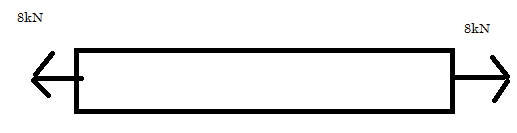# How to calculate average stress?

hello!

In the question below, is the average normal force in order to calculate the average normal stress, 8sin60 or 16sin60 or 0?

https://www.physicsforums.com/attachments/stress-jpg.100364/

thanks!

Might be just me, but i can't open that image.

OK the free body diagram is this;What do you think it is

I think it's zero, because at that point, the two opposite forces are cancelling each other

berkeman
Mentor
I think it's zero, because at that point, the two opposite forces are cancelling each other
The stress is not zero because there are forces pulling the bar apart.

SteamKing
Staff Emeritus
Homework Helper
I think it's zero, because at that point, the two opposite forces are cancelling each other
Ask this guy if the stress is zero:•Nidum, Chestermiller and billy_joule
ok then it's 16sin60

SteamKing
Staff Emeritus